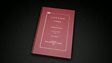Learn how to convert a denary number into a binary number using the binary place values method, with a worked example. The first thing to do is write the place values in their columns. Then find the largest place value number that fits into your denary number and subtract it. Put a one below and take the remainder to the next column. If the new number cannot be subtracted, put a zero in that column. Keep doing this until you have your binary number.This clip is from:
Bitesize

This clip could be used as a whole class introduction to the topic of converting denary numbers to binary. Encourage students to try the process on their own, using other denary numbers. This could be used during a lesson to support students through independent learning. This would also be useful as part of revision on data representation for GCSE students.